# How to Divide in ExcelClaudia Buckley#### Join the Excel conversation on Slack

Ask a question or join the conversation for all things Excel on our Slack channel.

Division is one of the four basic arithmetic operations that form the basis for other, more complex calculations. The common way to divide in Excel is to use the slash (/) as the division symbol. The format of the division formula in Excel is:

``=dividend / divisor``

where the number being divided is the dividend , and the divisor is the number being used to do the division. The operation 100 ÷ 25 on a calculator would be

````=100/25 `
```

in Excel.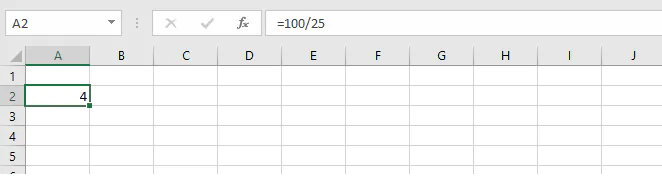A space may be placed between each element, but that’s not necessary. As with other formulas, you can use explicit values as shown above, or you can refer to cells containing the values to be used with cell references.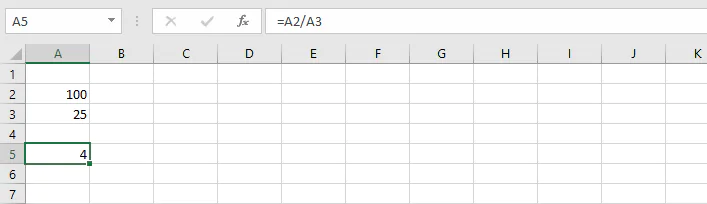The cell which contains the formula will display the result of the formula, but the Formula Bar will display what was actually typed into the cell.

## Divide function in Excel

Is there a divide function in Excel? You may have seen the QUOTIENT function and assumed that it was an alternative way to do division. You would have only been partially right. The QUOTIENT function is a way to divide, but only integers are returned, that is, the whole number without the remainder.

### QUOTIENT function

The syntax of the QUOTIENT function is

``=QUOTIENT(numerator, denominator)``

Here is an example of how it works.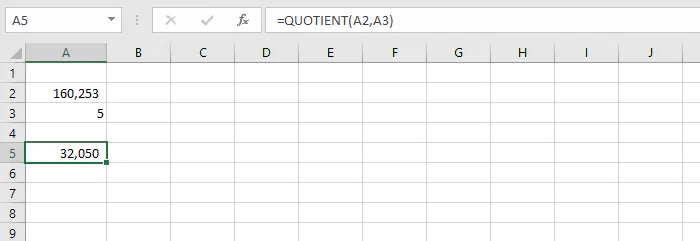In this example, we have asked Excel to divide the value 160,253 by 5 using the QUOTIENT function. If we had used the standard division formula, the result would have been 32,050.6. Since we used QUOTIENT, however, only the whole number - 32,060 - was returned, and the remainder discarded. This can be useful in circumstances where only whole numbers are valid, and rounding is not desired.

### MOD function

The flip side of the QUOTIENT function is the MOD function, which returns the remainder rather than the integer when one number is divided by another. The syntax is similar to the QUOTIENT function.

``=MOD(number, divisor)``

Applying the MOD function to our previous example, we would enter:

``=MOD(A2, A3)``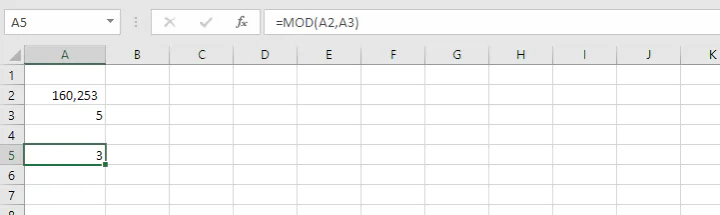We can tell from this formula that the remainder is 3, which amounts to 0.6

## Divide columns in Excel

Dividing values in one column by values in another column in Excel is quite easy. Take for instance, the situation below. We have a list of 6 spices where the brand name is shown in column A, the price in column B, and the volume of each container in column C. We can find out which product is most economical by dividing the price by the number of grams in each bottle.

In cell D2, we would enter:

``=B2/C2``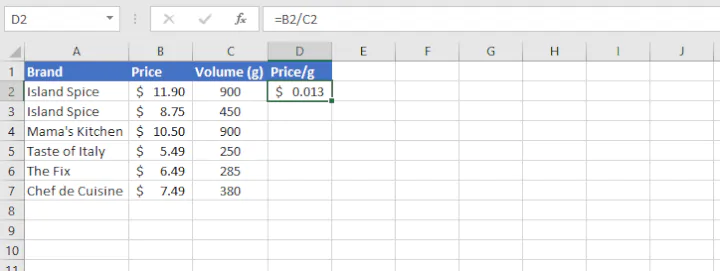We see here that the price per gram is determined to be \$0.013. (The number format was increased to three decimal places for reasons which you will soon see.)

To perform the same division operation on all the remaining rows without typing them in one by one, we can quickly copy the formula in cell D2 by either dragging or clicking the fill handle in the lower right corner of the active cell.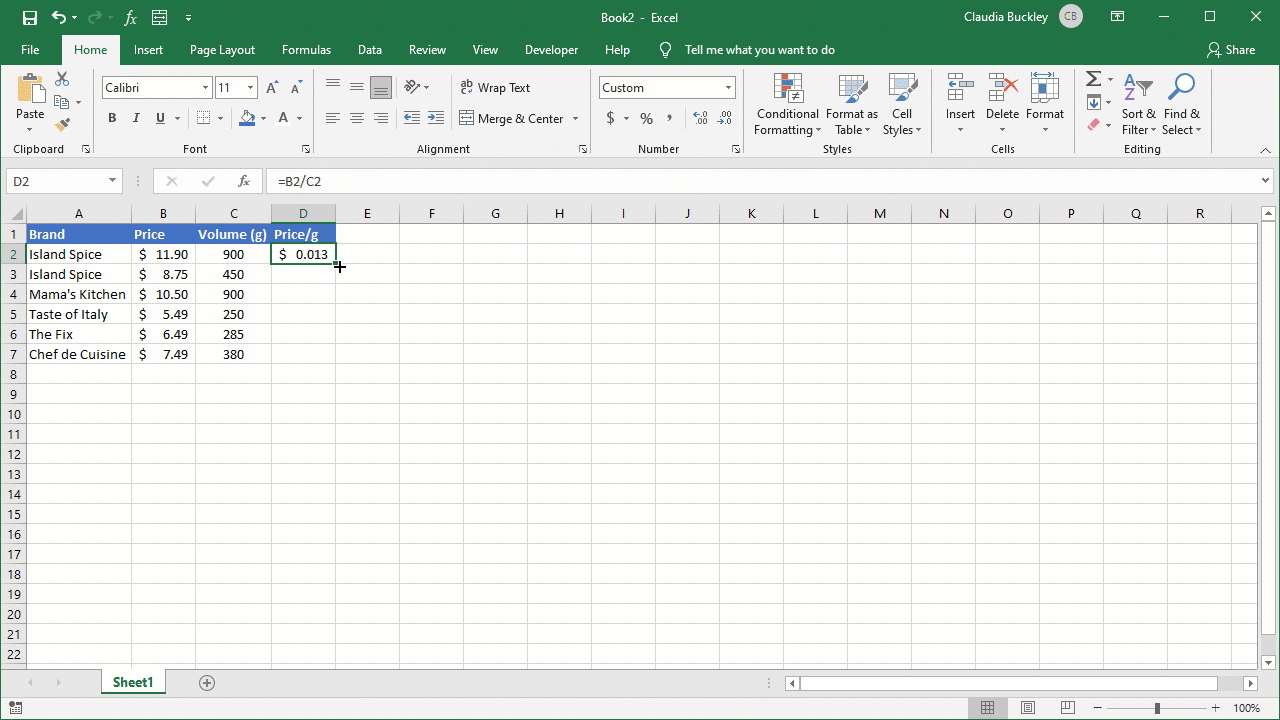Thanks to relative cell references, the formula adjusts for each row so that the formula in D3 divides B3 by C3, the one in D4 divides B4 by C4, and so on.

When our task is accomplished, we can see that each product works out to roughly 1 or 2 cents per gram, but increasing decimals to three places allows us to determine that the “Mama’s Kitchen” brand is the cheapest, at \$0.012 per gram, as shown in cell D4.

### How to divide one column by another using an array formula

An alternative to the above is to use an array formula (also known as CSE formula) to get the same result. We might prefer to use an array formula to prevent accidental deletion or alteration of a formula. This is because only the uppermost cell (the one where the formula was actually entered) in array formulas can be edited.

Here’s how to do it:

1. Select the entire range where you want to enter the formula (D2:D7 in this case).
2. Type =B2:B7/C2:C7 in cell D2 or in the Formula Bar.
3. Press Ctrl+Shift+Enter instead of Enter. If you’re using a Mac, press Command+Enter.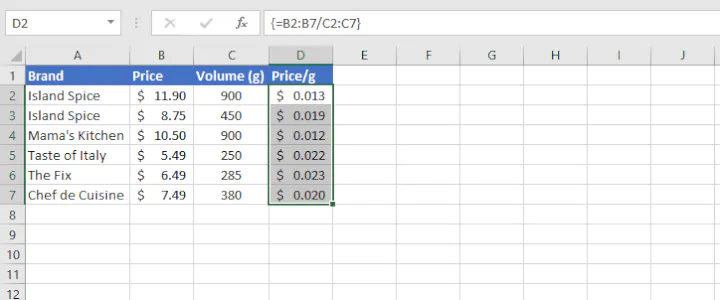Excel automatically encloses array formulas in curly brackets to remind you in the future that this was entered as a CSE formula.

### Divide a range of cells by a constant number

You can divide a range by a constant number either by entering that number as an explicit value (hardcoding it) within the formula, or by placing that constant value in a cell and using absolute cell referencing to divide by the value in that cell.

We can apply this to the following example where a restaurant menu has its prices listed in Canadian dollars (CAD). Using an exchange rate of \$1.25, we can calculate the prices in US dollars (USD) by dividing each value by 1.25.

#### Method 1 - Using a hardcoded divisor

Type the divisor directly into the formula as shown below:

``=B4/1.25``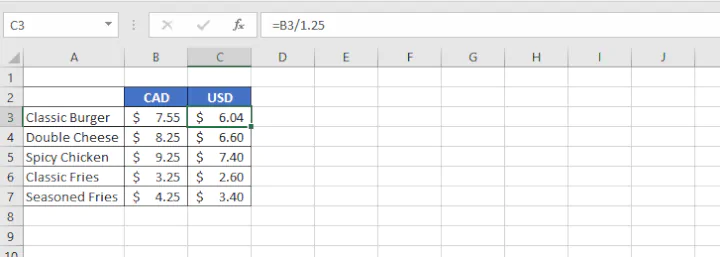We can copy the formula in C3 to the remaining cells as previously demonstrated to get the prices for the other items on the menu.

#### Method 2 - Using an absolute cell reference

An alternative (and often preferred) method is to enter the divisor in a location on the worksheet, and use that cell reference as the divisor.

The most important thing to remember at this stage is to ensure that the reference to that cell does not shift when the formula is copied to other cells. We do this by “fixing/locking” or making the reference absolute by placing a dollar sign before the column name and before the row number.

``=B4/\$B\$1``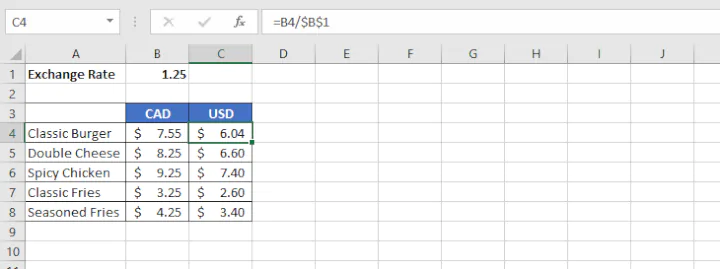Copying the formula in this way means that all the prices in column B will be divided by whatever value is entered in cell B1.

The advantage with this method is that if we choose to update our exchange rate, only one cell needs to be changed, and all the currency conversions will be updated automatically.

## DIV/0 error when dividing in Excel

If you ever encounter an error response that says #DIV/0!, this points to the fact that you attempted to divide by zero, or by an empty cell.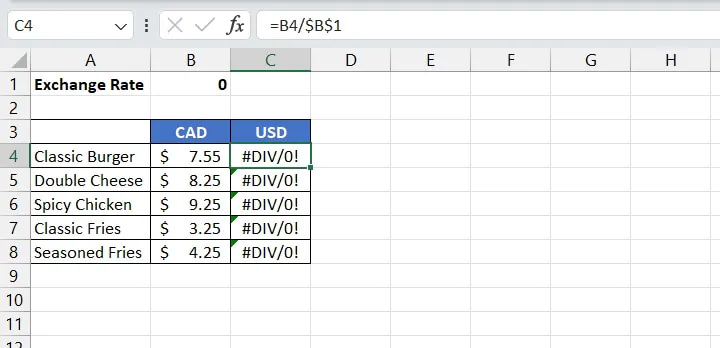Sometimes this is unavoidable because you’re waiting on some other information to enter the divisor. If you’re like me and you don’t like seeing errors on your worksheet, you may consider using an IFERROR formula to catch the error and return an alternative result.

``=IFERROR(B4/\$B\$1, “”)``

This formula tells Excel to display a blank if the calculation results in an error.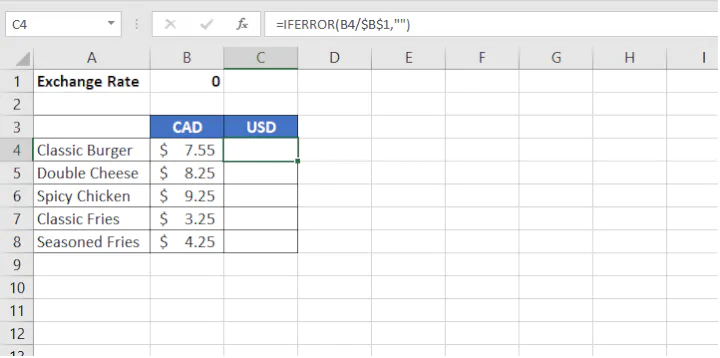Become a certified Excel ninja with GoSkills bite-sized courses#### Join the Excel conversation on Slack

Ask a question or join the conversation for all things Excel on our Slack channel.Claudia Buckley

Claudia is a project manager and business skills instructor at GoSkills. In her spare time, she reads mystery novels and does genealogy research.